Function Repository Resource:

# EnhancedPlot

Plot with several enhancements added for singularities, asymptotes, surd values and non-interval domains

Contributed by: Dennis M Schneider
 ResourceFunction["EnhancedPlot"][f,{x,xmin,xmax}] returns a plot with several enhancements given by options.

## Details and Options

The most significant options are "Asymptote", "Exception", "FindExceptions", "FindAsymptotes", "PowerBehavior" and "ZeroToNull".
Vertical asymptotes can be plotted by setting the "Asymptote" option to a list of x coordinates at which there are infinite (possibly one-sided) discontinuities.
Point discontinuities can be plotted by setting the "Exception" option to a list of x coordinates where possible exceptions occur. Removable discontinuities are denoted by an empty circle. Jump discontinuities are denoted by one or more empty circles at the points of discontinuity (the "jump points"). Infinite discontinuities are drawn as vertical asymptotes. A filled circle is drawn at all other points.
Set "FindExceptions" to True to use ResourceFunction["FunctionDiscontinuities"] to find the discontinuities.
Set "FindAsymptotes" to True to use ResourceFunction["Asymptotes"] to find the various types of asymptotes: "Horizontal", "Vertical", "Oblique", "Parabolic" or "Other".
Set "PowerBehavior" to Real (the default) to insure that odd roots of negative real numbers are returned as negative real numbers instead of the imaginary numbers. Set "PowerBehavior" to Complex to restore the Wolfram Language’s default behavior.
Set "ZeroToNull" to True (the default) to change the Wolfram Language’s default behavior of assigning the value 0 when none of the conditions given in the piecewise function apply to assigning the value Null. Set "ZeroToNull" to False to restore default behavior.
"PointStyle" will not accept color directives.
ResourceFunction["EnhancedPlot"] accepts the following options for adding enhancements:
 "Asymptote" {} list of points where the function has vertical asymptotes "AsymptoteStyle" {{Red,Dashed}} graphics directive to specify the style for each asymptote "DrawPoint" {} list of points to add to the plot "DrawPointStyle" AbsolutePointSize graphics directives to specify style(s) for the points given with "DrawPoint" "Exception" {} list of points where the function has discontinuities "FindExceptions" Automatic whether to use ResourceFunction["FunctionDiscontinuities"] to find the discontinuities "HorizontalAsymptote" {} list of points where horizontal asymptotes should be drawn "HorizontalAsymptoteStyle" {{Red,Dashed}} graphics directive to specify the style for each horizontal asymptote "PointStyle" AbsolutePointSize graphics directive to specify the style for the point(s) where the function has a discontinuity; color directives are not allowed "PowerBehavior" Real odd roots of negative real numbers are returned as negative real numbers "ZeroToNull" True whether to change the default value of a piecewise function from zero to Null
In addition to these options, ResourceFunction["EnhancedPlot"] accepts any of the options for Plot, as well as the option "ExcludeRemovableDiscontinuities" for the resource function FunctionDiscontinuities.

## Examples

### Basic Examples (8)

Find the discontinuities and plot a function:

 In:=Out=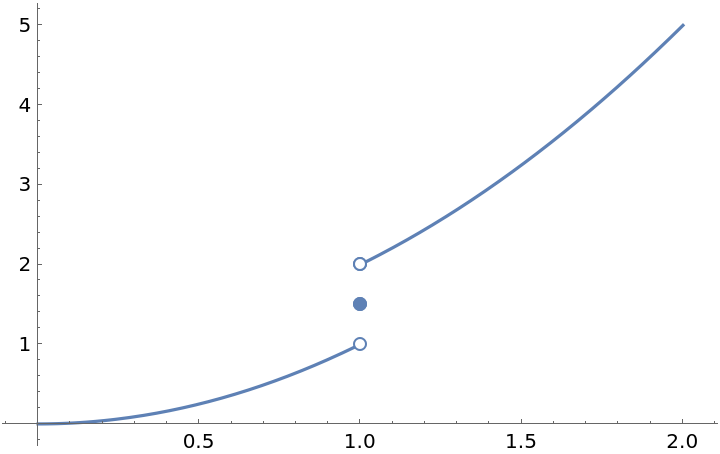Explicitly give the discontinuity:

 In:=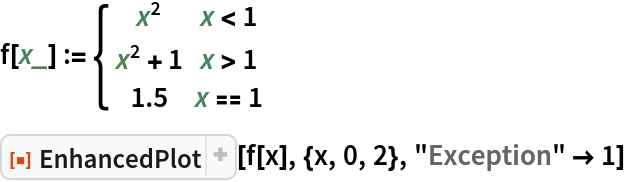Out=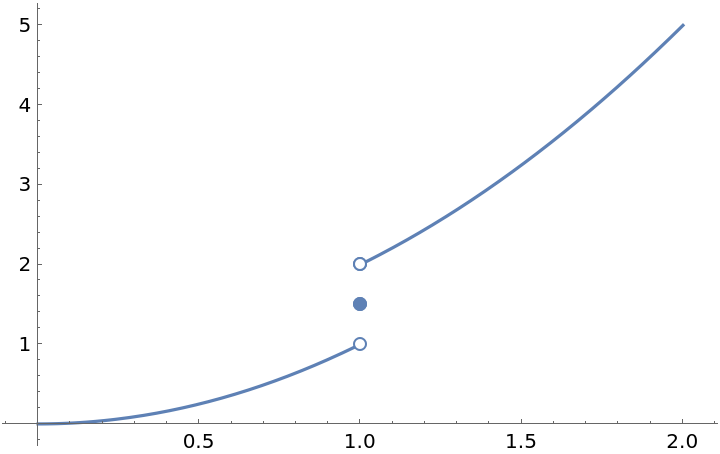Plot a function with a removable discontinuity:

 In:=Out=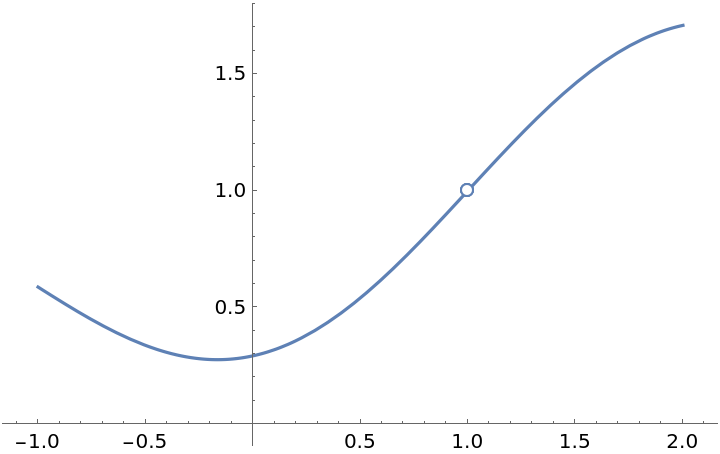Explicitly give the discontinuity:

 In:=Out=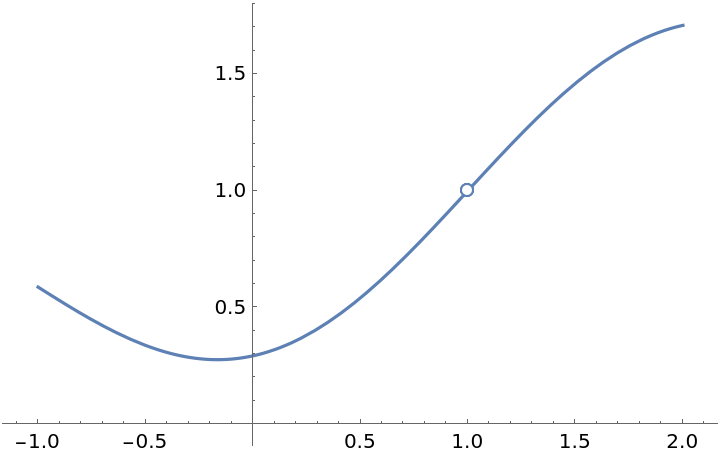Remove the discontinuity:

 In:=Out=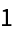In:=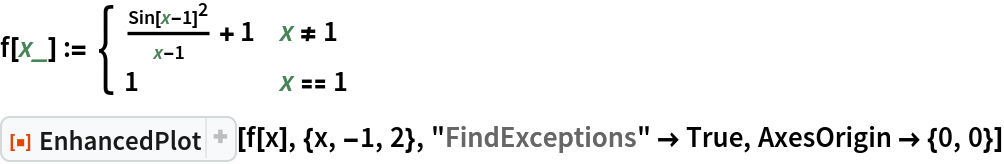Out=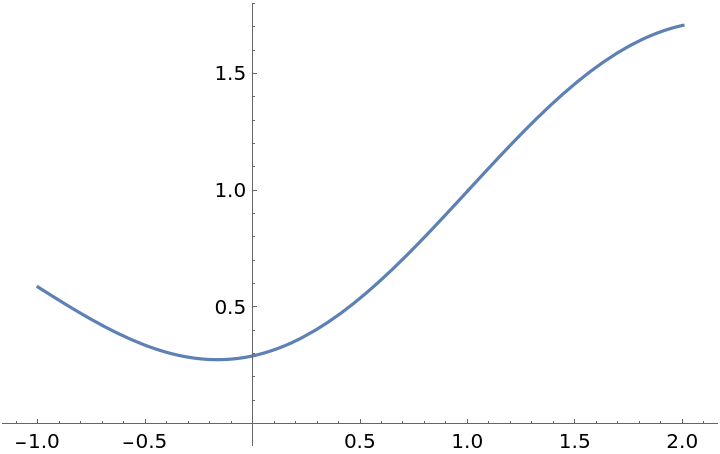Remove the discontinuity but show the break point:

 In:=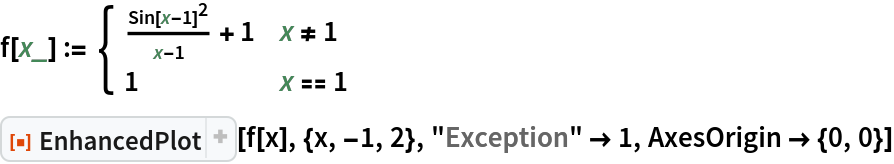Out=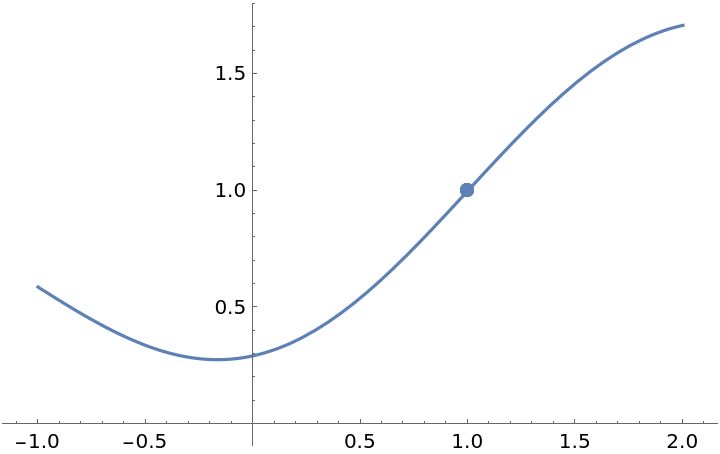Plot a function with an asymptote at x=-1, a horizontal asymptote at y=0, a jump discontinuity at x=2, and a removable discontinuity at x=3:

 In:=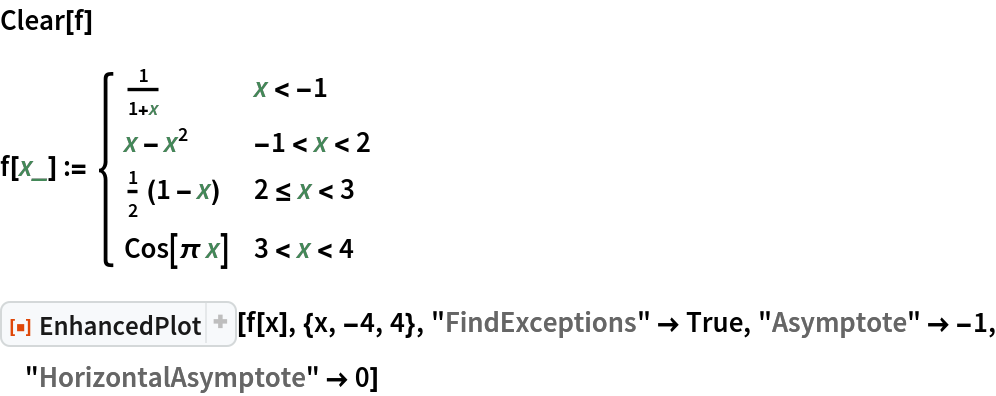Out=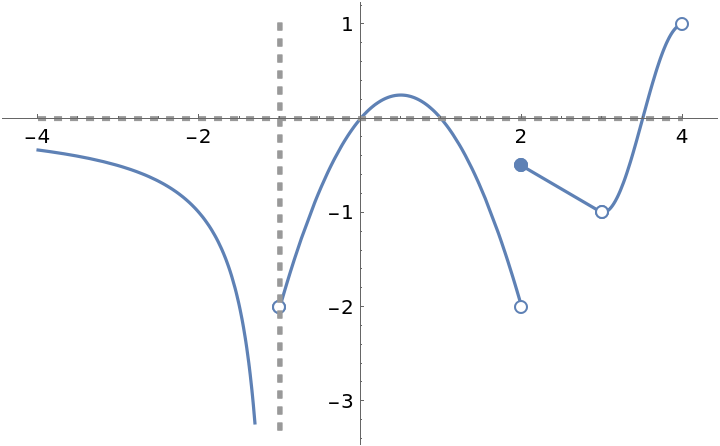"FindAsymptote" misses the horizontal asymptote at y=0:

 In:=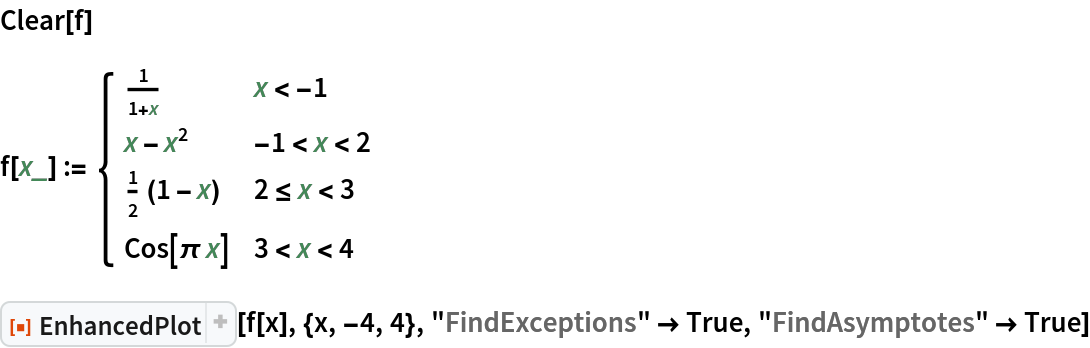Out=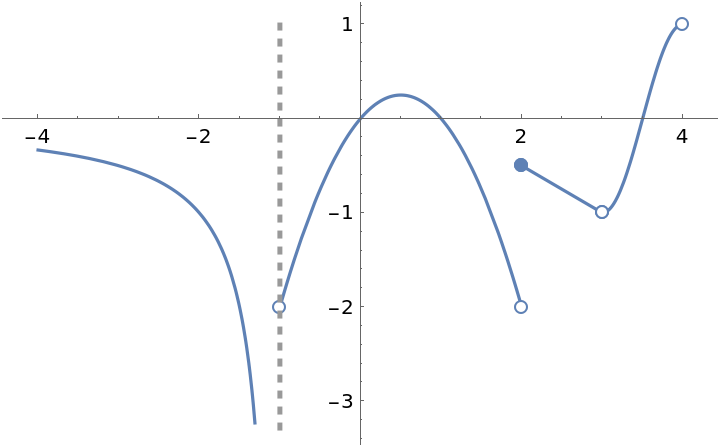An example from the resource function FunctionDiscontinuities:

 In:=Out=Plot the result using the Plot command and the option ExclusionsStyle:

 In:=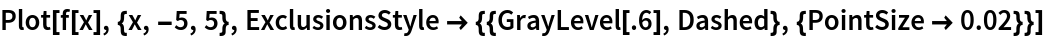Out=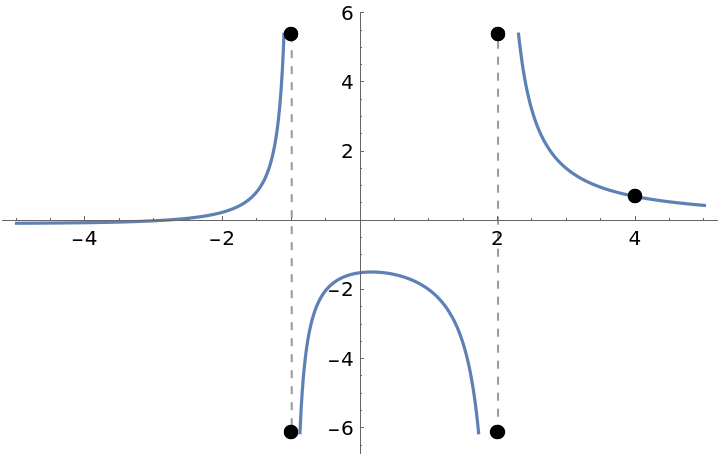Use EnhancedPlot to obtain a much better plot:

 In:=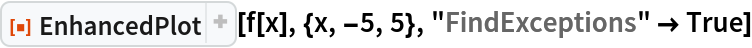Out=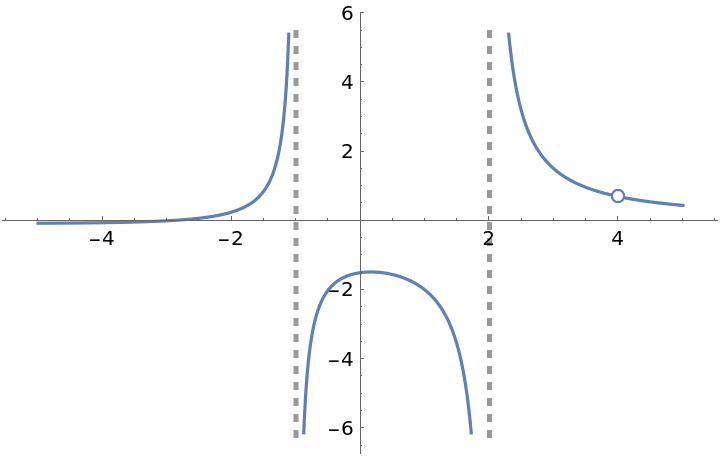It is not necessary to call on the resource function Asymptotes to find the vertical asymptotes of the following function since they are among the discontinuities found by the resource function FunctionDiscontinuities:

 In:=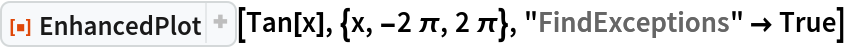Out=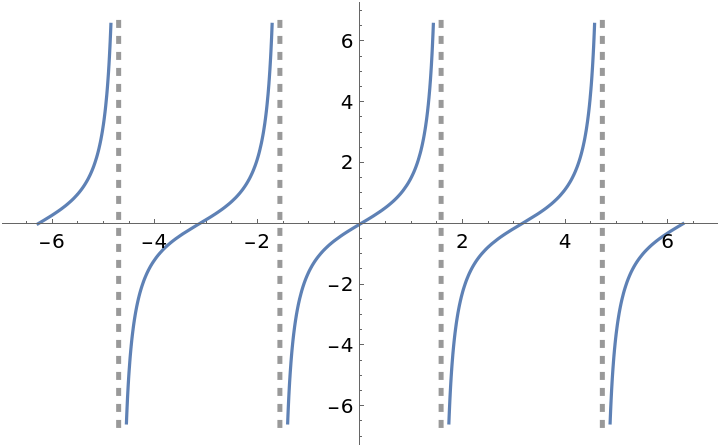Plot a function with asymptotes and a removable discontinuity:

 In:=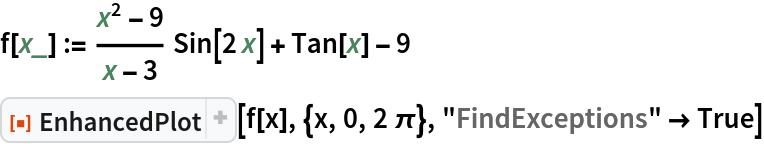Out=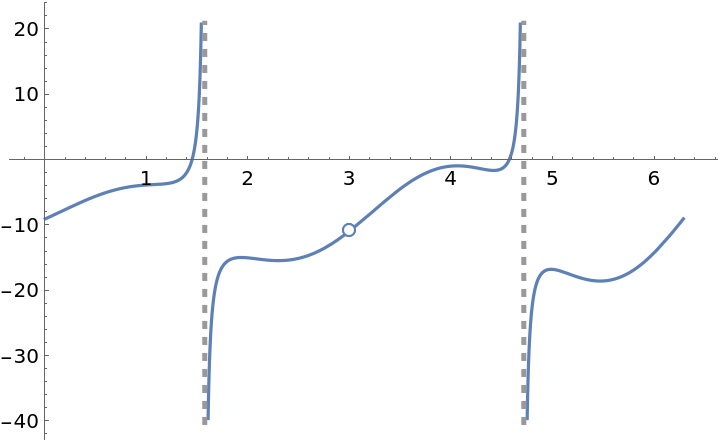In:=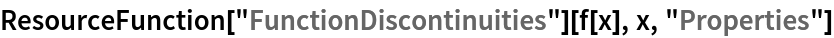Out=Remove the singularity by appropriately defining the function at x=3 so that f(3)=limn3f(x):

 In:=Out=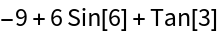In:=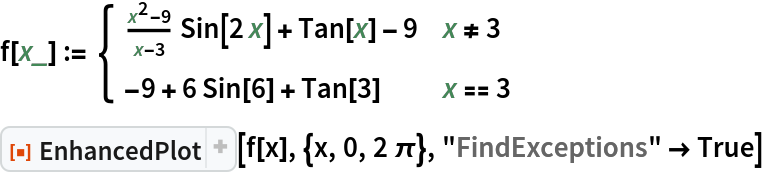Out=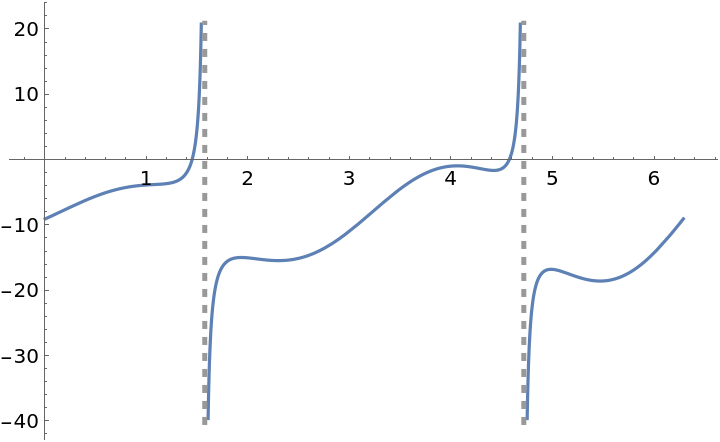Explicitly list the jump point and add the asymptotes manually:

 In:=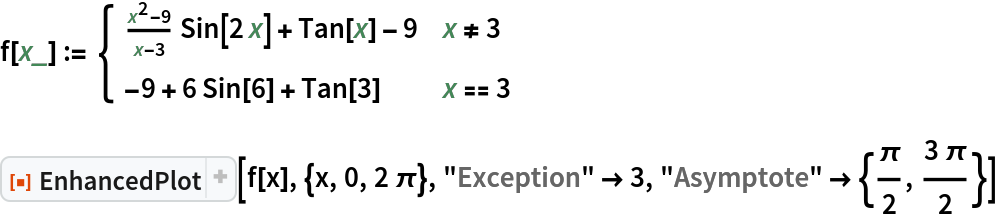Out=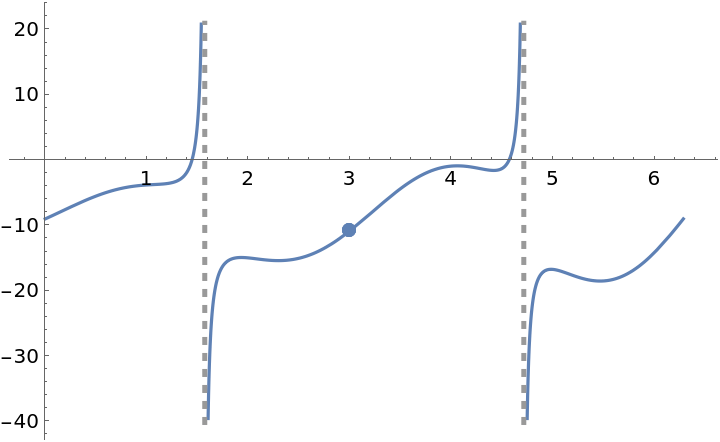Plot a function with a jump discontinuity:

 In:=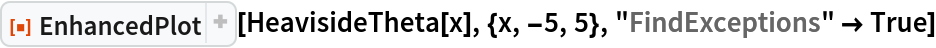Out=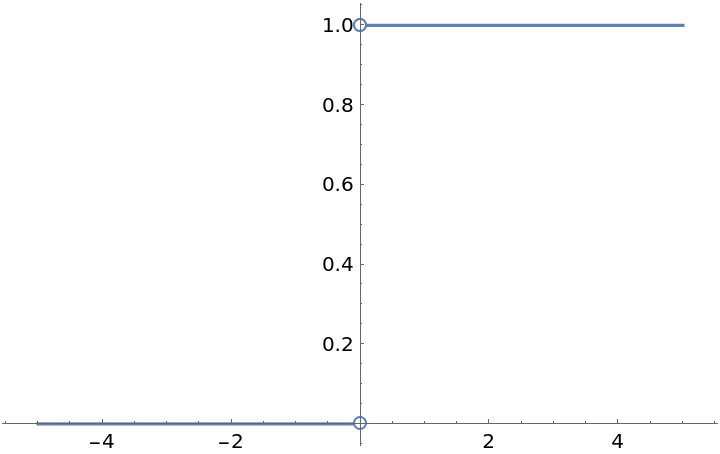A function with an odd root over a domain consisting of two disjoint intervals with one interval containing negative numbers. Plot the function and its derivative:

 In:=Out=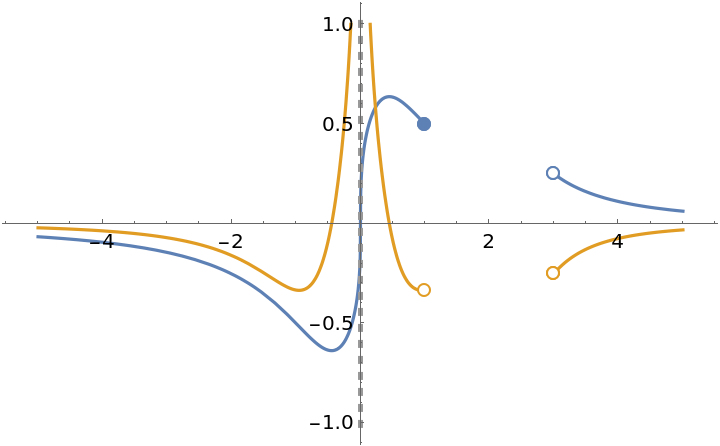### Options (17)

#### Asymptote and AsymptoteStyle (1)

Plot a function with two vertical asymptotes and apply a style to the asymptotes:

 In:=Out=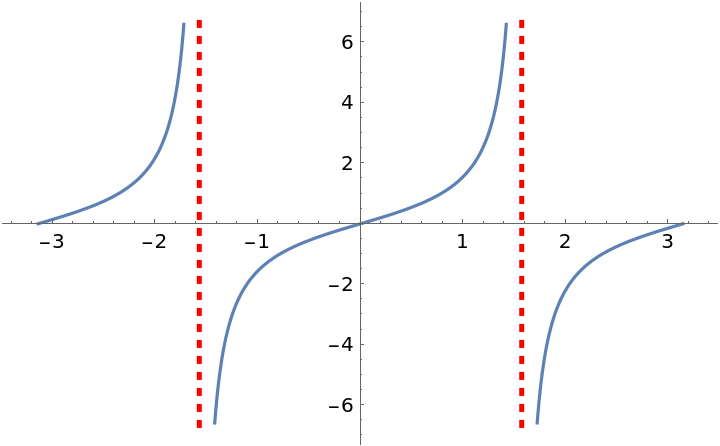#### DrawPoint and DrawPointStyle (2)

Plot a curve with a removable discontinuity and two points drawn off the curve:

 In:=Out=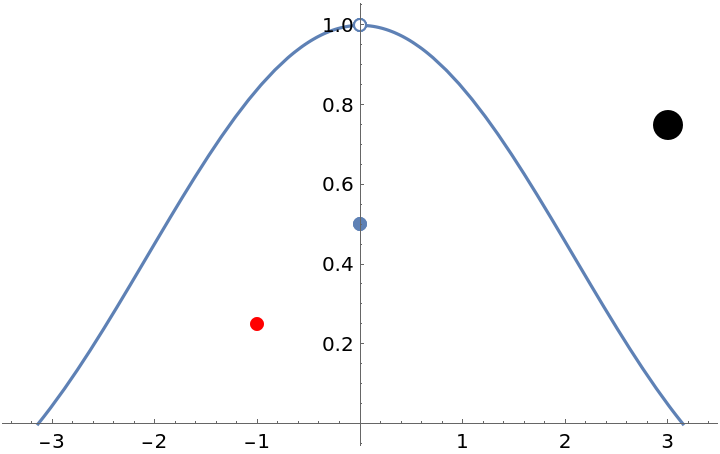Plot a curve with a removable discontinuity and several points drawn on the curve:

 In:=Out=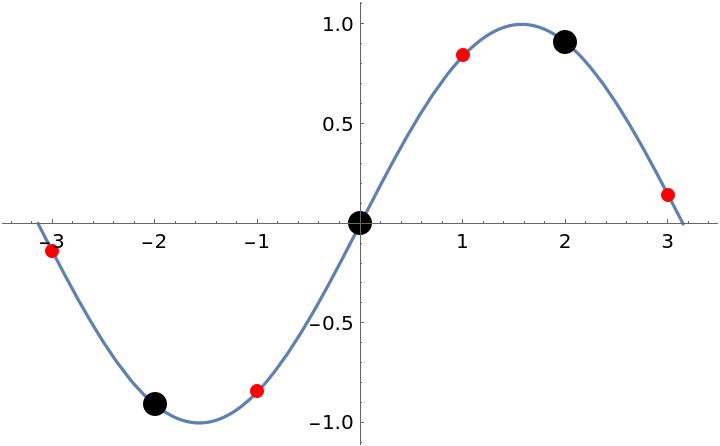#### Exception (1)

Create plot with several exceptions; a removable discontinuity (at x=-1/2), a jump discontinuity (at x=-1) and an infinite discontinuity (at x=1):

 In:=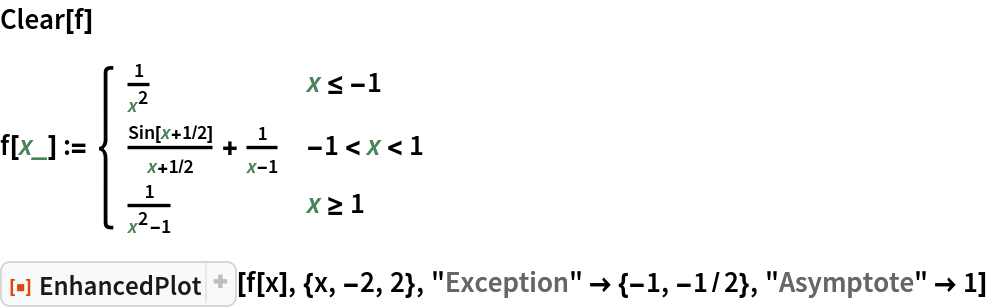Out=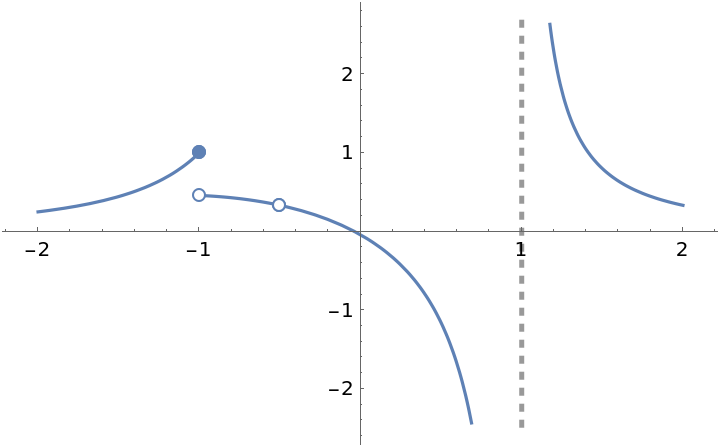#### FindAsymptotes (2)

Setting "FindAsymptotes"True uses the resource function Asymptotes to find the asymptotes (one vertical and one oblique) of the following function:

 In:=Out=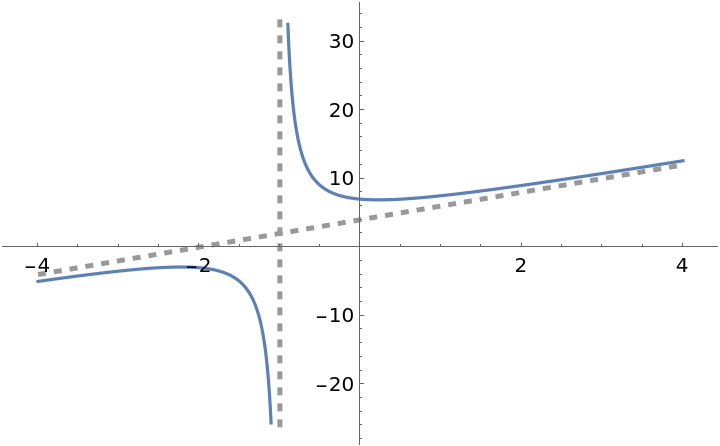Plot a function with asymptotes (vertical and other):

 In:=Out=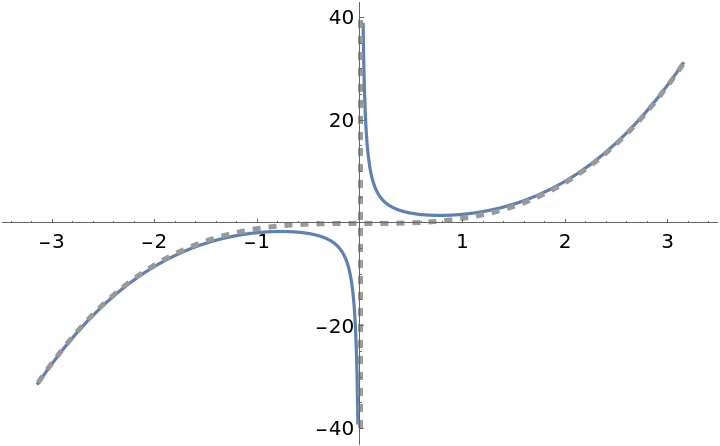#### FindExceptions (3)

Setting "FindExceptions"True uses the resource function FunctionDiscontinuities to find the discontinuities:

 In:=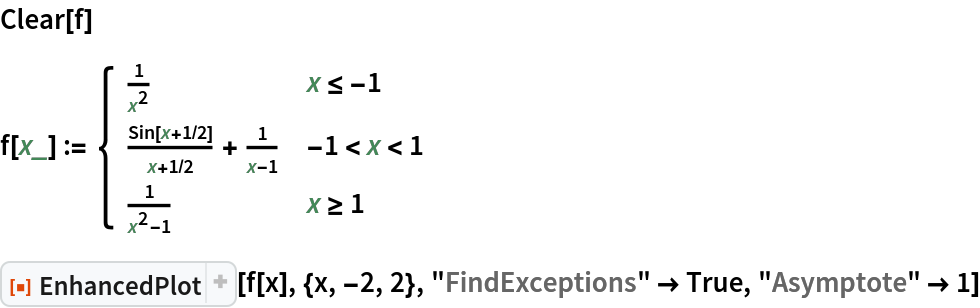Out=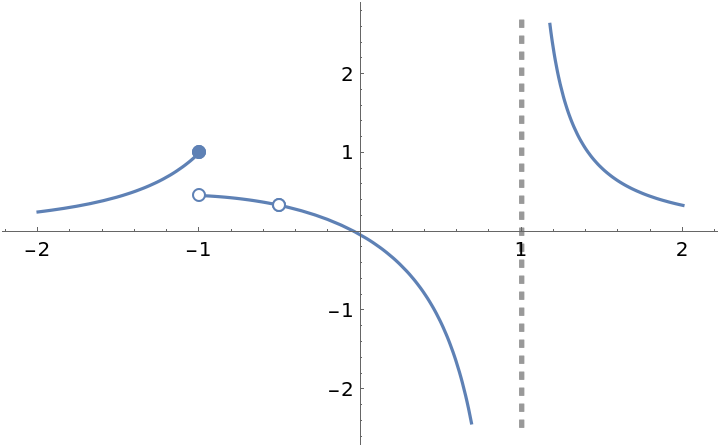Plot two functions:

 In:=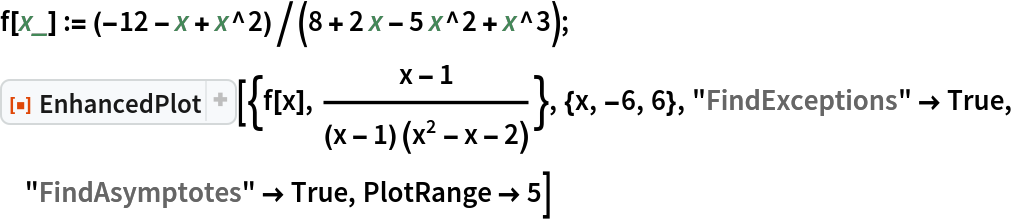Out=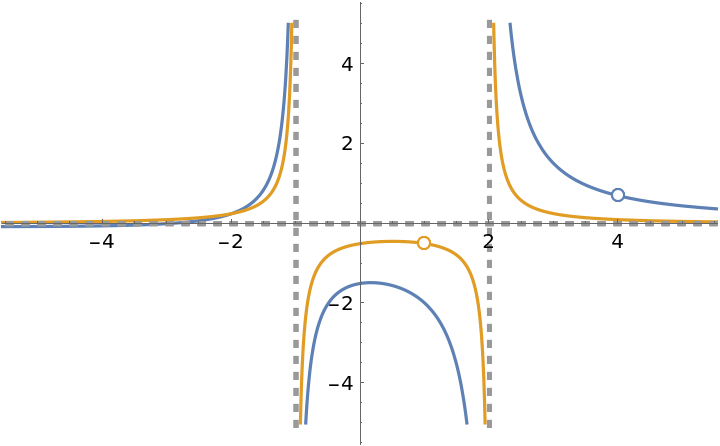If a point is listed as an exception but isn’t an exception, then a solid point is plotted on the graph:

 In:=Out=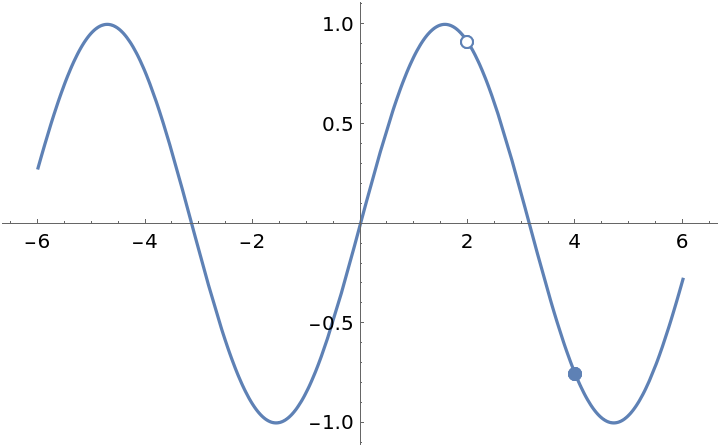#### HorizontalAsymptote and HorizontalAsymptoteStyle (1)

Plot a function with its horizontal asymptote:

 In:=Out=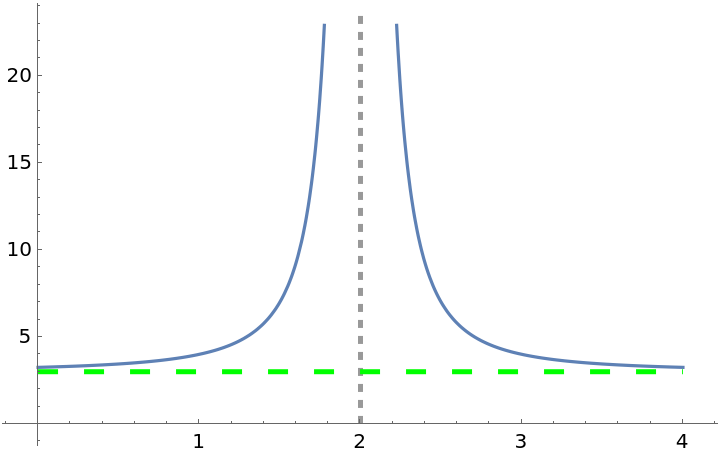In:=Out=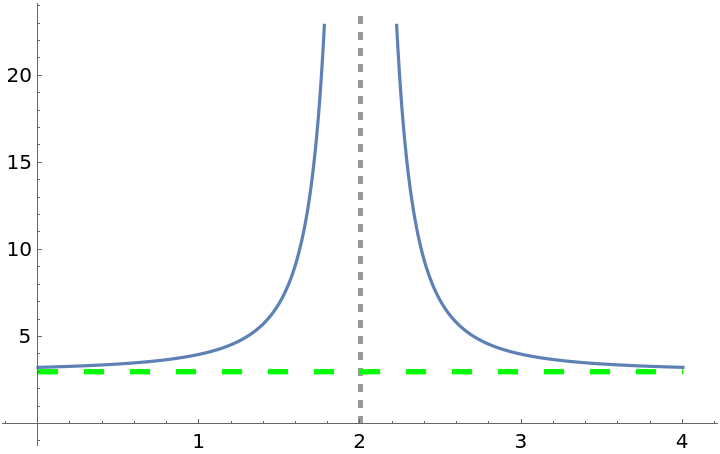#### PointStyle (1)

Make the points bigger:

 In:=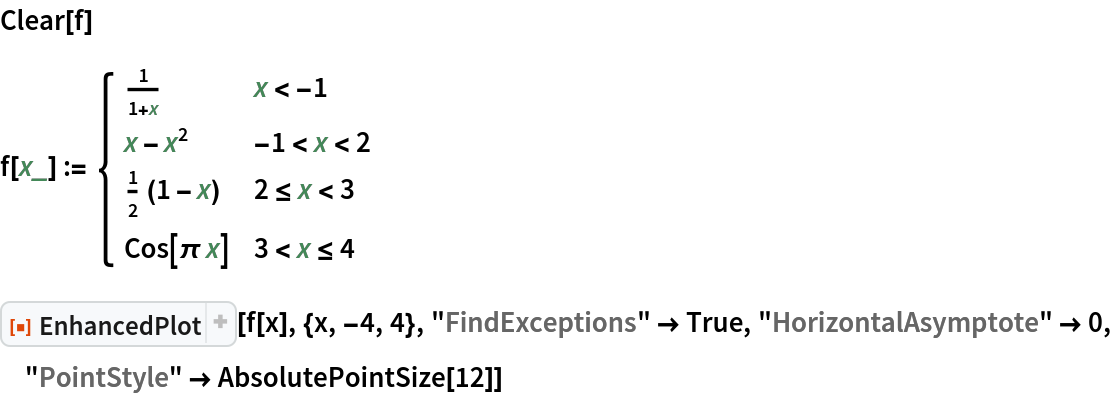Out=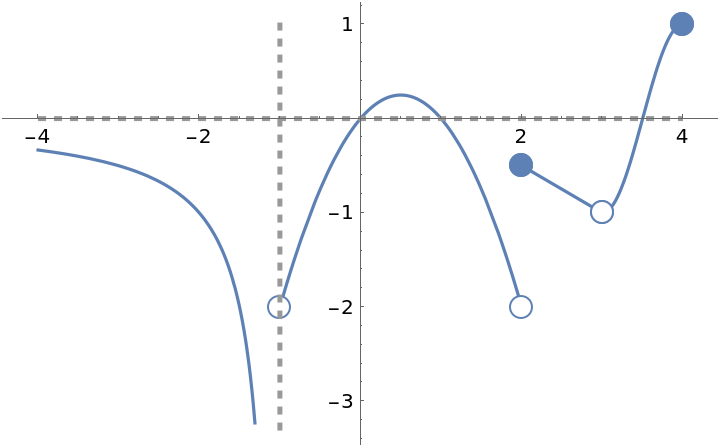In:=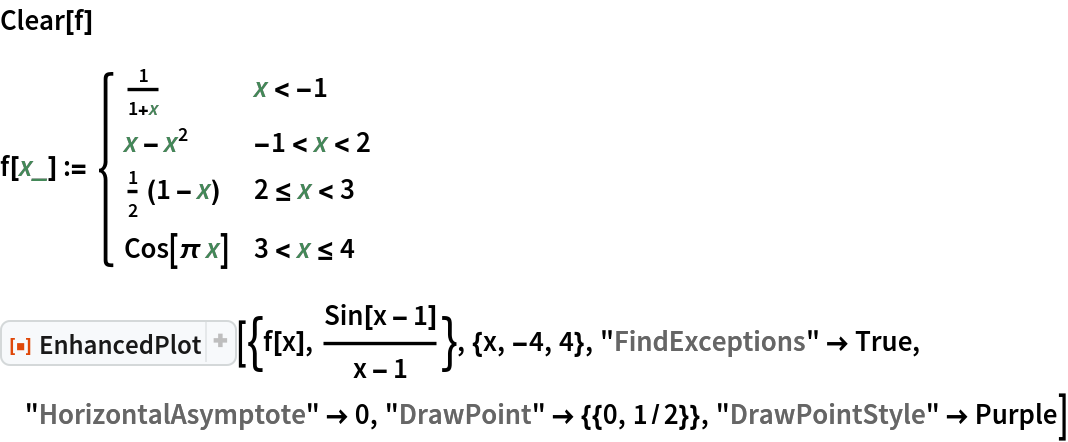Out=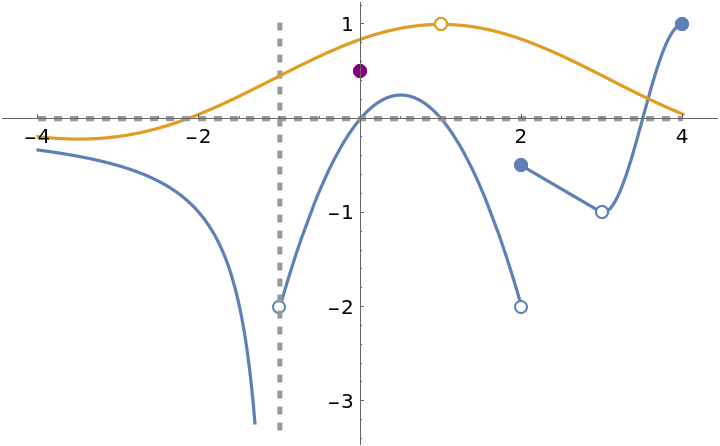#### PowerBehavior (1)

By default, the Wolfram Language returns the principal root of a number. If the number is real and negative and the root is odd, then the principal root is an imaginary number. Setting "PowerBehavior" to Real (the default) will force EnhancedPlot to plot the positive root:

 In:=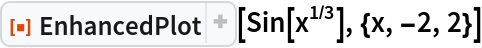Out=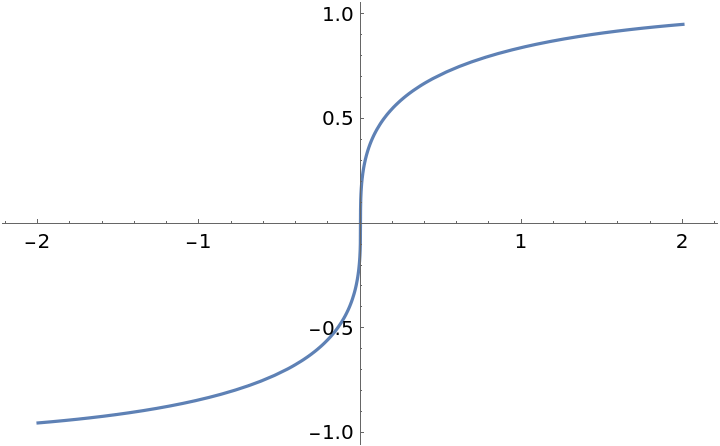#### ZeroToNull (5)

By default, the Wolfram Language does not produce a correct plot of a piecewise function whose domain consists of disjoint intervals. The reason is that it uses 0 as the default value if none of the conditions apply:

 In:=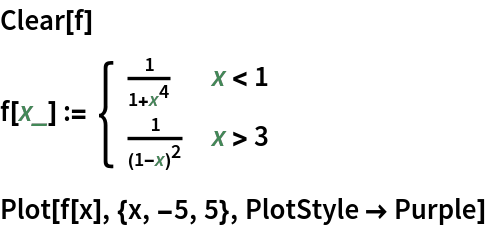Out=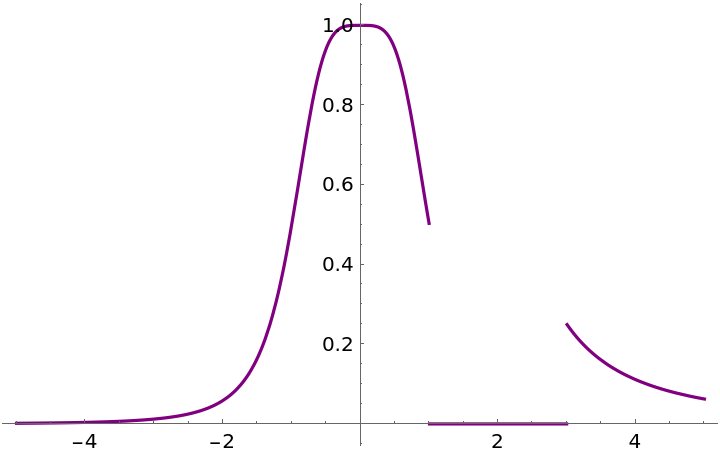A correct plot is obtained by manually setting the default value of the Piecewise to Null:

 In:=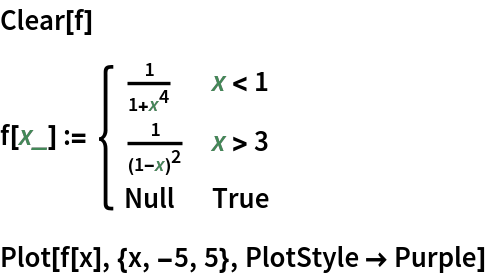Out=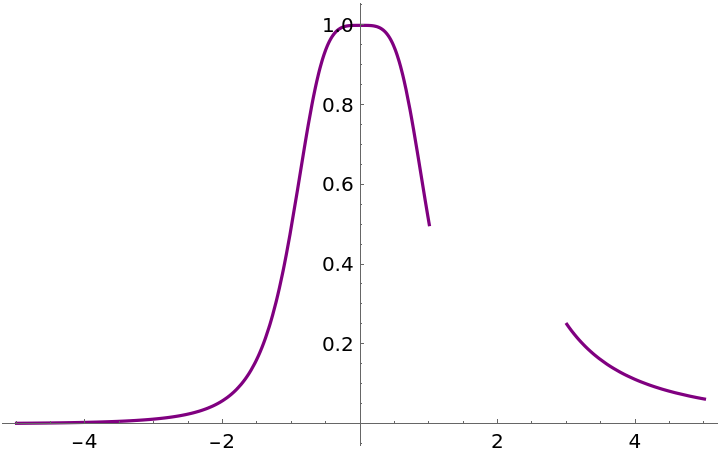EnhancedPlot automatically sets the default value of Piecewise to Null:

 In:=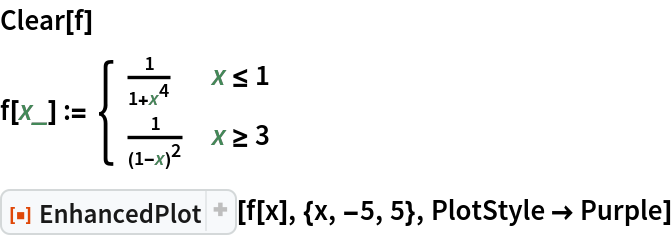Out=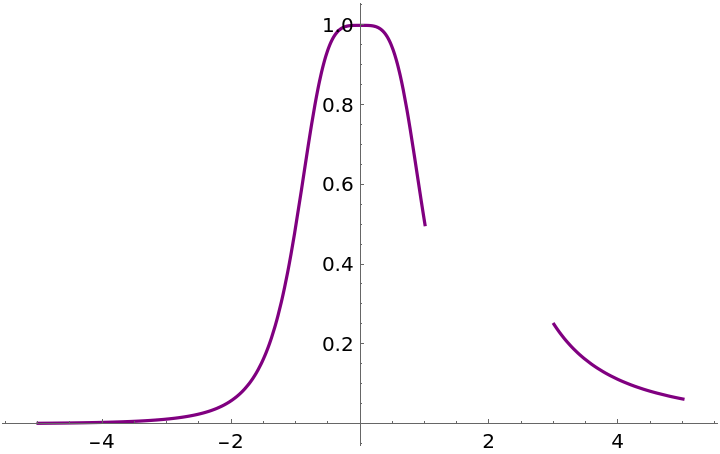Return default Plot behavior by setting the option "ZeroToNull" to False:

 In:=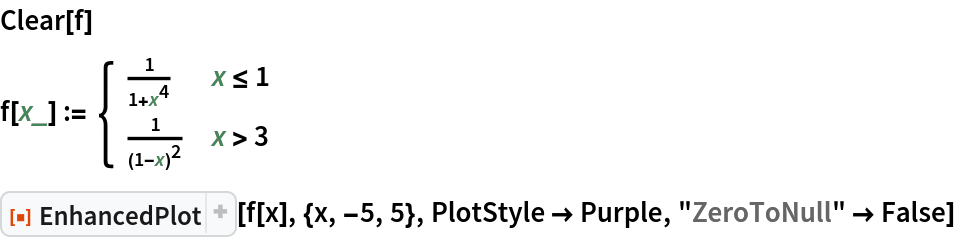Out=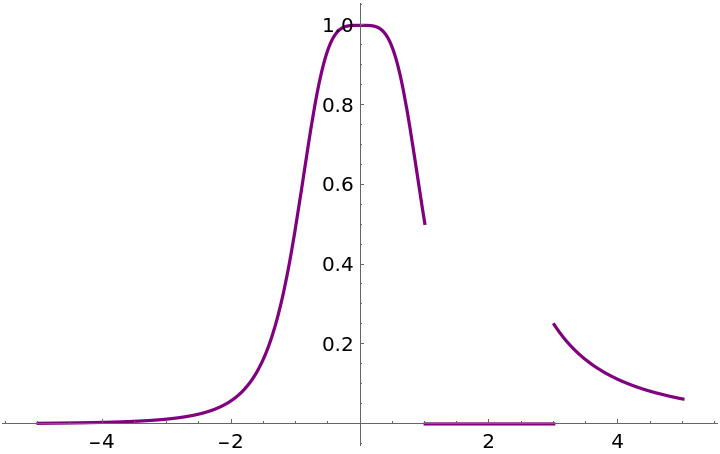Note that with the option "ZeroToNull" set to False, "FindExceptions" will list the break points as jump discontinuities:

 In:=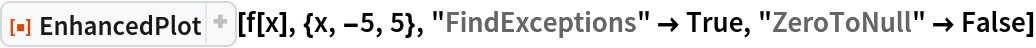Out=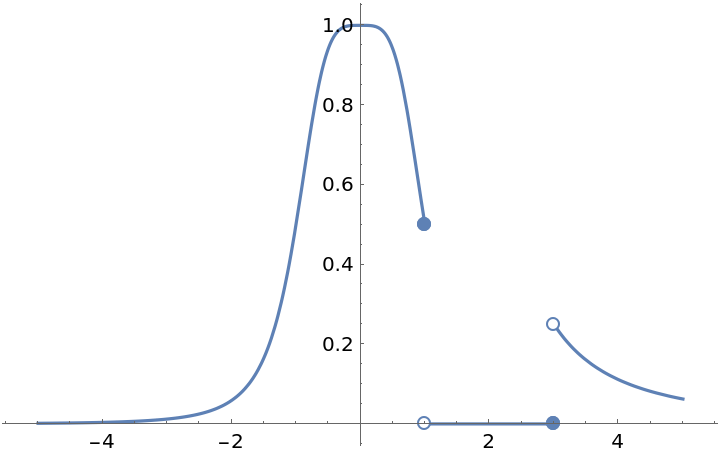### Possible Issues (4)

The resource function Asymptotes can be very slow:

 In:=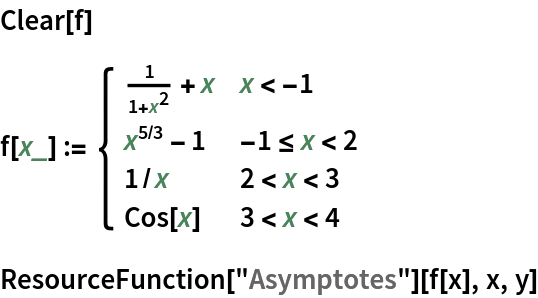Out=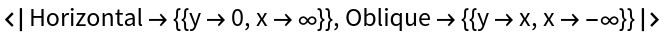This contributes a significant amount of time to EnhancedPlot if the setting "FindAsymptotes"True is used:

 In:=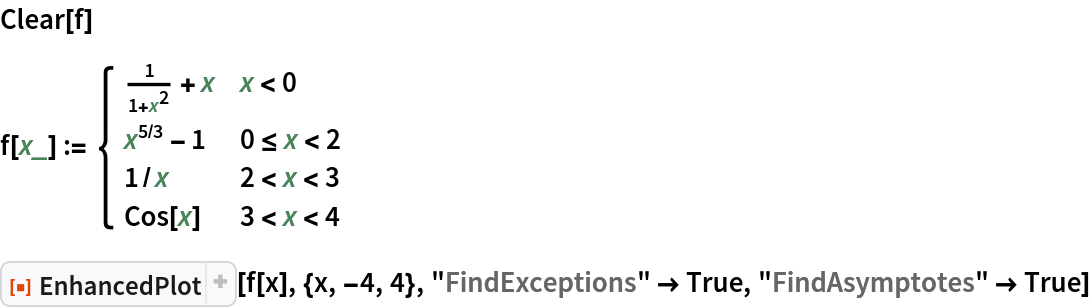Out=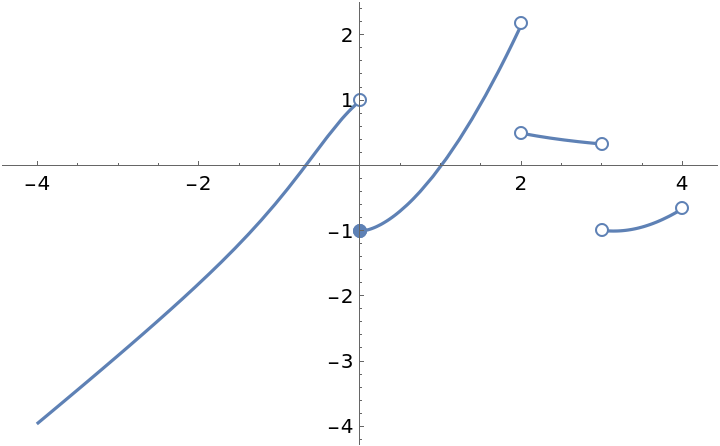On the other hand, "FindExceptions" is very fast:

 In:=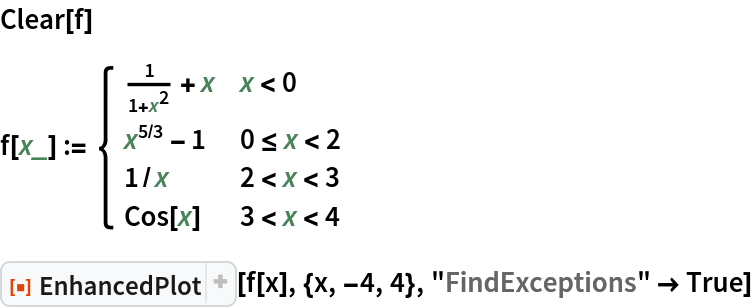Out=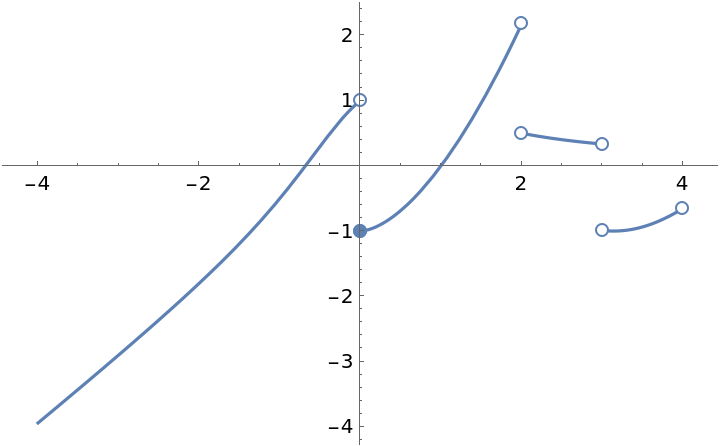The resource function Asymptotes can miss the asymptotes of some functions:

 In:=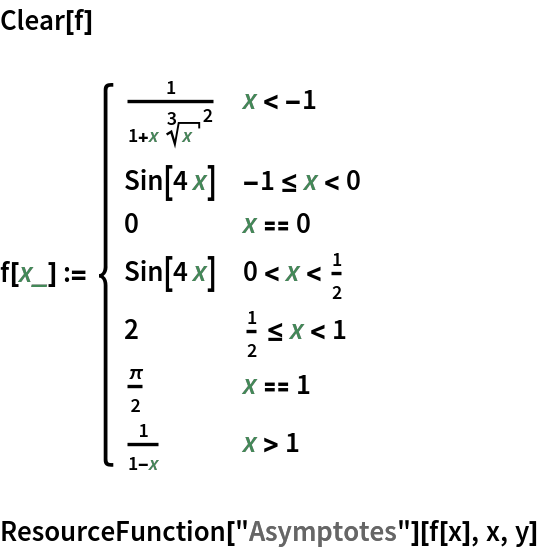Out=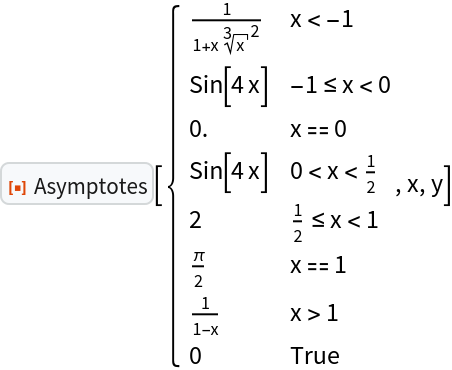The resource function FunctionDiscontinuities will find the exceptions:

 In:=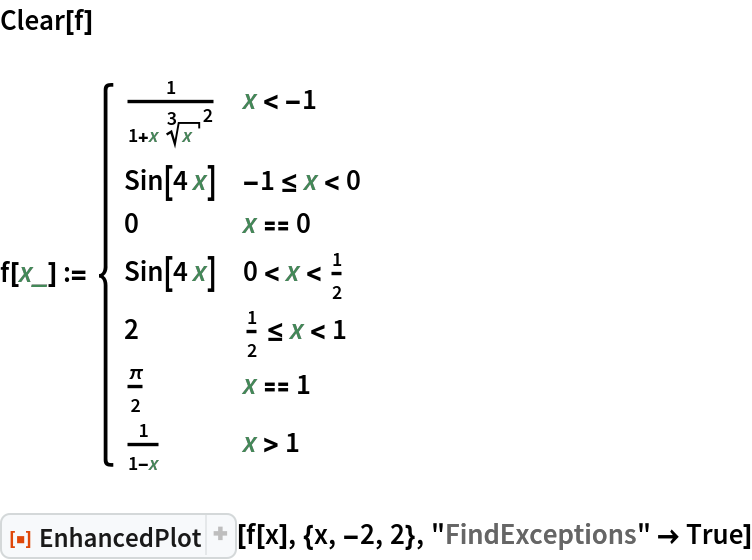Out=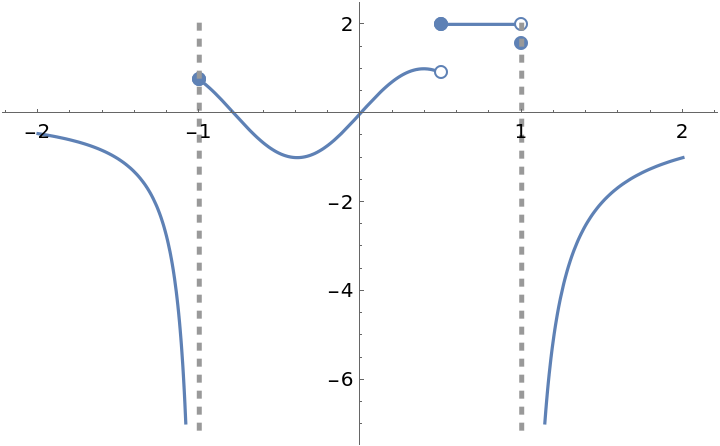EnhancedPlot sometimes misses a removable discontinuity:

 In:=Out=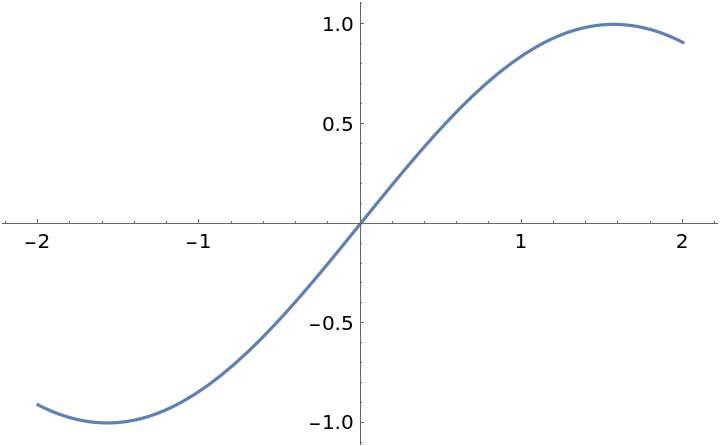However, the discontinuity can be listed manually:

 In:=Out=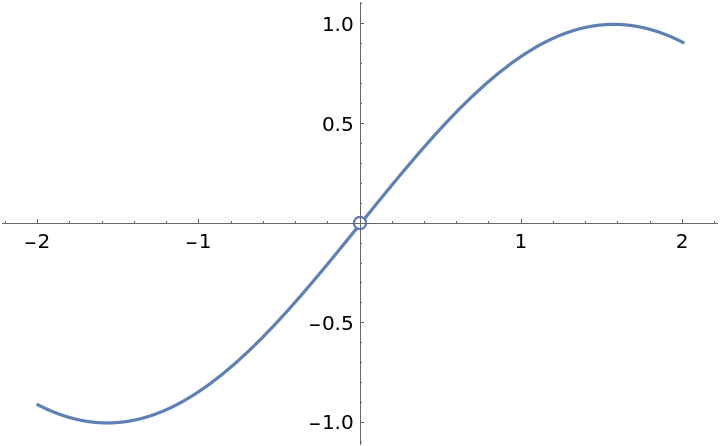When a discontinuity denoted by a filled circle is overlapped by a empty circle, the filled circle is covered by the empty circle. That is, the empty circle is not really empty (see behavior at x=4):

 In:=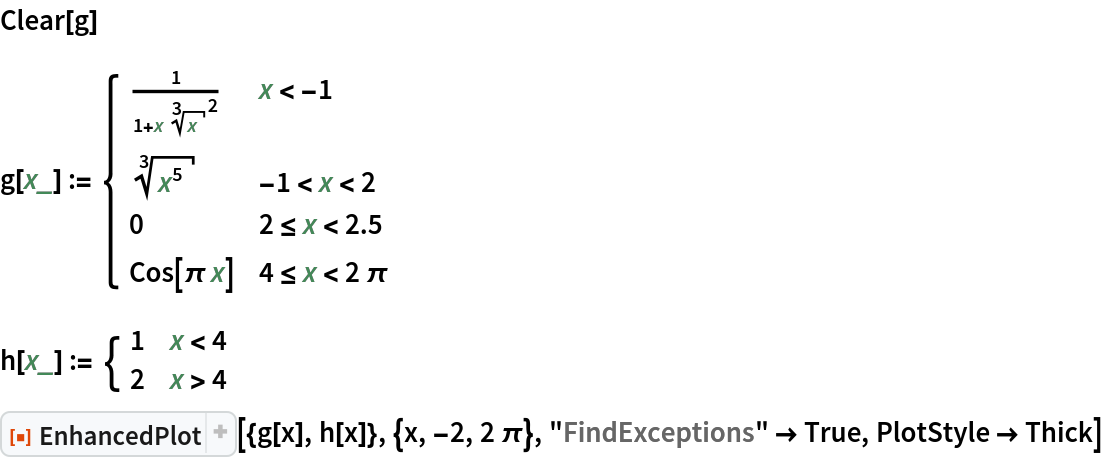Out=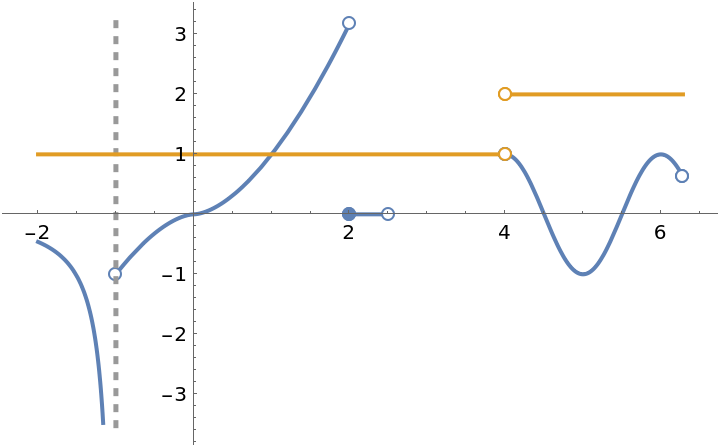## Publisher

Dennis M Schneider

## Version History

• 2.1.1 – 06 September 2022
• 2.1.0 – 09 May 2022
• 2.0.0 – 21 August 2020
• 1.0.0 – 06 December 2019